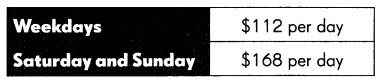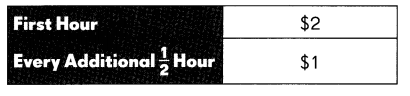# Math in Focus Grade 5 Chapter 2 Practice 8 Answer Key Real-World Problems: Multiplication and Division

Go through the Math in Focus Grade 5 Workbook Answer Key Chapter 2 Practice 8 Real-World Problems: Multiplication and Division to finish your assignments.

## Math in Focus Grade 5 Chapter 2 Practice 8 Answer Key Real-World Problems: Multiplication and Division

Question 1.
Rafael has 118 baseball cards arranged in an album. Each page of the album can hold 9 cards.
How many pages are full and how many cards are on the last page?
Total 14 pages,
Last page has one card,

Explanation:
Rafael has 118 baseball cards arranged in an album.
Each page of the album can hold 9 cards.
118  ÷  9 = 13 + R 1,
Total 14 pages,
Last page has one card.

Question 2.
A ski club had 146 members. Each member paid $30 a month for training fees. How much did the club collect in fees for the year? Answer:$365,

Explanation:
A ski club had 146 members,
Each member paid $30 a month for training fees. 146 x 30 = 4380, 4380 ÷ 12 = 365, So, the club collect in fees for the year =$365.

Question 3.
A farmer collects 1,250 eggs on a morning. She puts 30 eggs on each tray.
How many egg trays does she need to hold all the eggs?
42 egg trays,

Explanation:
A farmer collects 1,250 eggs on a morning.
She puts 30 eggs on each tray.
Total egg trays she need to hold all the eggs
1250 ÷ 30 = 41 + R 20,
42 egg tray needed.

Question 4.

At a supermarket, pineapple juice sells at $1 per pint (16 ounces). Greg wants to buy eighteen 40-ounce cans of pineapple juice from the supermarket. How much does he have to pay altogether? Answer:$45,

Explanation:
At a supermarket, pineapple juice sells at $1 per pint (16 ounces). Greg wants to buy eighteen 40-ounce cans of pineapple juice from the supermarket. 1 pint = 16 ounces =$1,
18 x 40 = 720 ounces,
720 ÷ 16 = $45. Solve. Show your work. Question 5. A charitable organization spends$4,500 giving out food vouchers to families.
a. Each family receives one voucher worth $25. How many families are there? Answer: 180 families, Explanation: A charitable organization spends$4,500 giving out food vouchers to families.
Each family receives one voucher worth $25. Total number of families$4,500 ÷ $25 = 180. b. Each voucher will be worth$32 next year. How much more money will the charity need next year?
$1,260, Explanation: A charitable organization spends$4,500 giving out food vouchers to families.
Total number of families = 180,
Each voucher will be worth $32 next year 180 x$32 =  $5760,$5,760 – $4,500 =$1,260.

Question 6.
A group of tourists visits an art museum. The admission is $13 for each adult and$7 for each child.
There are 10 adults and 18 children in the group. How much do they pay altogether?
$256, Explanation: The admission is$13 for each adult and $7 for each child. There are 10 adults and 18 children in the group.$13 x 10 = $130,$7 x 18 = $126,$130 + $126 =$256 they pay altogether.

Question 7.
The length of a rectangular board is 10 centimeters longer than its width.
The width of the board is 26 centimeters. The board is cut into 9 equal pieces.

a. What is the area of each piece?
104 square cm,

Explanation:
The length of a rectangular board is 10 centimeters longer than its width.
The width of the board is 26 centimeters.
The board is cut into 9 equal pieces.
width = w,
length = l = w + 10cm,
width = 26 cm
length = 36 cm
total area = 26  x 36 = 936 cm2
936 ÷ 9 = 104 square cm.

b. What are the possible dimensions of each piece?
(Take the dimensions to be whole numbers.)
13 X 8 or  8 x 13 possible dimensions of each piece,

Explanation:
From the above information,
length X width = 104 square cm,
13 x 8 = 104 = 8 x 13 possible dimensions of each piece.

Question 8.
There are 912 yellow chairs and blue choirs altogether in an auditorium.
The blue chairs are arranged in 36 rows with 12 chairs in each row.
The yellow chairs are arranged in rows of 20. How many rows of yellow chairs are there?
24 rows,

Explanation:
912 yellow and  blue chairs in the auditorium.
The blue chairs are arranged in 36 rows with 12 chairs in each row.
The yellow chairs are arranged in rows of 20.
number of yellow rows of yellow chairs are
36 x 12 = 432 blue chairs
912 – 432 = 480
480 ÷ 20 = 24 rows.

Question 9.
The table shows the wages of workers in Samantha’s company.
Sean works from Tuesday through Sunday each week. How much does he earn in 1 week?$784, Explanation: Sean works from Tuesday through Sunday each week. The wages of weekdays 4 x$112 =$448, Wages of Saturday and Sunday 2 x$168 = $336, Total amount he earn in 1 week$448 + $336 =$784.

Question 10.
The table shows the charges at a parking garage.a. Sharona parked her car at the garage from 9:30 A.M. to 11:00 A.M. on the same day.
How much did she have to pay?
$3, Explanation: First hour the parking charges =$2,
Every additional half an hour = $1, Sharona parked her car at the garage from 9:30 A.M. to 11:00 A.M. 9:30 to 11:00 = 1 and half hour is parking time,$2 + $1 =$3.
$7, Explanation: First hour the parking charges =$2,
Every additional half an hour = $1, Daryll parked his car there from 9 A.M. to 12:30 P.M, 9 AM to 12:30 AM = 3 hours and 30 minutes, =,3 x$2 + $1 =$6 + $1 =$7.